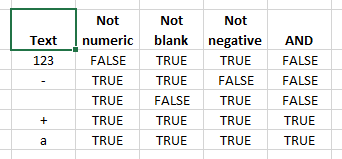# VBA TextBox number validation

A demonstration of the code logic for the xlf Multiplication Program - TextBox number validator.

## Number PreFilter - the VBA code

Code 1: Sub procedure NumberValidator demonstrates the code logic of a TextBox prefilter
Sub NumberValidator()

Dim TestArray(1 To 5) As String
Dim ReturnArray(1 To 5, 1 To 4) As Boolean
Dim ACell As Range

Dim i As Integer
Dim Temp As String

Set ACell = ActiveCell

TestArray(1) = "123"
TestArray(2) = "-"
TestArray(3) = ""
TestArray(4) = "+"
TestArray(5) = "a"

For i = LBound(TestArray) To UBound(TestArray)
Temp = TestArray(i)
ReturnArray(i, 1) = Not IsNumeric(Temp)
ReturnArray(i, 2) = Temp <> ""
ReturnArray(i, 3) = Temp <> "-"
ReturnArray(i, 4) = Not IsNumeric(Temp) And Temp <> "" And Temp <> "-"
Next i

With ActiveCell
.Offset(0, 0) = "Text"
.Offset(0, 1) = "Not numeric"
.Offset(0, 2) = "Not blank"
.Offset(0, 3) = "Not negative"
.Offset(0, 4) = "AND"
End With

ActiveCell.Select
Selection.Resize(6, 1).Select
Selection.HorizontalAlignment = xlCenter
ACell.Select

For i = LBound(TestArray) To UBound(TestArray)
ActiveCell.Offset(i, 0).Value = TestArray(i)
ActiveCell.Offset(i, 1).Value = ReturnArray(i, 1)
ActiveCell.Offset(i, 2).Value = ReturnArray(i, 2)
ActiveCell.Offset(i, 3).Value = ReturnArray(i, 3)
ActiveCell.Offset(i, 4).Value = ReturnArray(i, 4)
Next i

Selection.Resize(1, 5).Select
With Selection
.HorizontalAlignment = xlCenter
.WrapText = True
.Font.Bold = True
End With

ACell.Select

End Sub


The output to the ActiveCell is provided in figure 1. A number, minus sign, and blank all return FALSE.

1.Run the private procedure from the VBE. Use F5 or Run > Run Sub on the menu.

• This example was developed in Excel 2013 and VBA 7.1
• Revised: Saturday 25th of February 2023 - 09:37 AM, [Australian Eastern Standard Time (EST)]